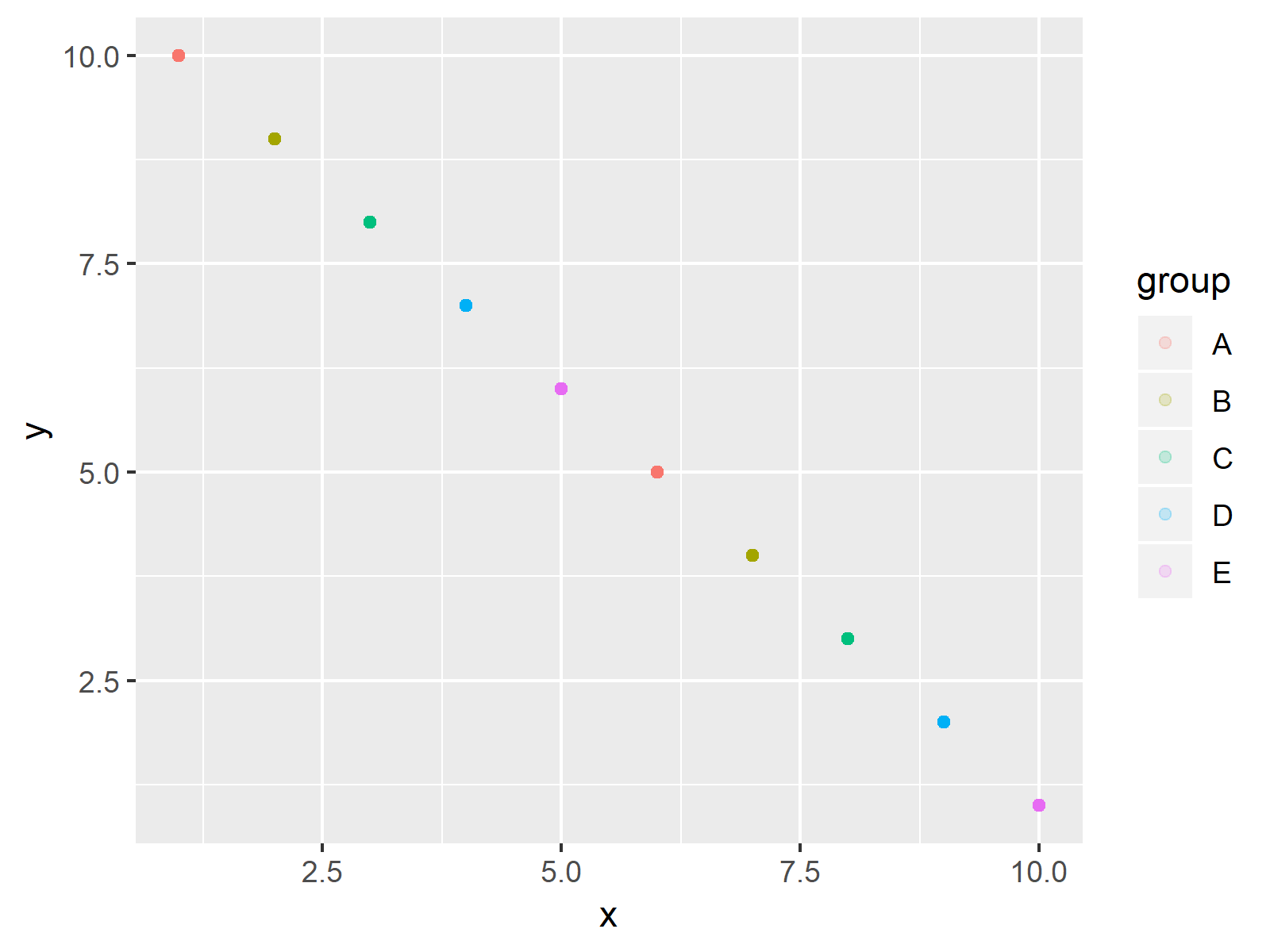# Set Legend Alpha of ggplot2 Plot in R (Example)

In this R tutorial you’ll learn how to adjust the transparency of ggplot2 legend items.

The article consists of one example for the modification of alpha values. More precisely, the tutorial looks as follows:

Let’s dive right into the R syntax.

## Example Data, Add-On Packages & Default Graphic

As a first step, we have to create some data that we can use in the following examples:

```data <- data.frame(x = 1:10,                     # Create example data
y = 10:1,
group = LETTERS[1:5])
data                                             # Print data
#     x  y group
# 1   1 10     A
# 2   2  9     B
# 3   3  8     C
# 4   4  7     D
# 5   5  6     E
# 6   6  5     A
# 7   7  4     B
# 8   8  3     C
# 9   9  2     D
# 10 10  1     E```

Have a look at the previous RStudio console output. It shows that our exemplifying data consists of ten rows and three columns. The variables x and y contain some numeric values and the variable group specifies the groups of our data points.

For the examples of this tutorial, we’ll also need to install and load the ggplot2 package.

```install.packages("ggplot2")                      # Install ggplot2 package

Now, we can draw our data with default legend items as follows.

```ggp <- ggplot(data, aes(x, y, col = group)) +    # Example plot
geom_point()
ggp                                              # Draw plot```As shown in Figure 1, the previous R programming syntax plotted a ggplot2 scatterplot with solid legend items (i.e. default alpha value).

## Example: Modifying Legend Alpha of ggplot2 Plot

The following R syntax illustrates how to decrease the alpha (i.e. to increase transparency) of our legend symbols. For this, we have to change the alpha value within the guide_legend function. Have a look at the following R code and the resulting graph:

```ggp +                                            # Adjust alpha argument
guides(colour = guide_legend(override.aes = list(alpha = 0.2)))```The output of the previous R programming syntax is shown in Figure 2: It shows our ggplot2 graphic with transparent legend symbols.

## Video & Further Resources

If you need further info on the R programming codes of this tutorial, you might want to have a look at the following video of my YouTube channel. In the video, I’m showing the R codes of this tutorial.

Please accept YouTube cookies to play this video. By accepting you will be accessing content from YouTube, a service provided by an external third party.If you accept this notice, your choice will be saved and the page will refresh.

In addition, I can recommend to read the other tutorials of my website. You can find some posts here.

In summary: At this point you should have learned how to increase or decrease the legend alpha of ggplot2 plots in the R programming language. In case you have further questions, please let me know in the comments below.

Subscribe to the Statistics Globe Newsletter